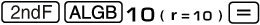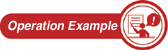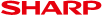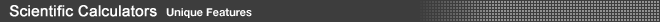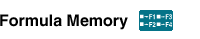Frequently used formulas or complex equations can be stored as functions in Formula Memory. By recalling the formula, you can substitute new variables into the equation and recalculate it without having to enter the entire sequence again. Since you can do repeat equations, it's also convenient for simulations.• How to Use It
(1) Store the equation into the calculator.
 You can put four equations into formula memory with the F1-F4 keys.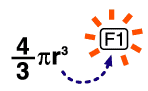Note: Even when the power is off, the equation will remain in memory until a new equation is stored.
(2) Call up the equation.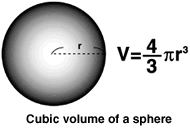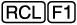(3) Use the equation to calculate.